Courses

# Test: Single Correct MCQs: Equilibrium | JEE Advanced

## 42 Questions MCQ Test Class 11 Chemistry 35 Years JEE Mains &Advance Past yr Paper | Test: Single Correct MCQs: Equilibrium | JEE Advanced

Description
This mock test of Test: Single Correct MCQs: Equilibrium | JEE Advanced for JEE helps you for every JEE entrance exam. This contains 42 Multiple Choice Questions for JEE Test: Single Correct MCQs: Equilibrium | JEE Advanced (mcq) to study with solutions a complete question bank. The solved questions answers in this Test: Single Correct MCQs: Equilibrium | JEE Advanced quiz give you a good mix of easy questions and tough questions. JEE students definitely take this Test: Single Correct MCQs: Equilibrium | JEE Advanced exercise for a better result in the exam. You can find other Test: Single Correct MCQs: Equilibrium | JEE Advanced extra questions, long questions & short questions for JEE on EduRev as well by searching above.
QUESTION: 1

### Molten sodium chloride conducts electricitry due to the presence of

Solution:

In molten state the cations and anions become free and flow of current is due to migration of these ions in opposite directions in the electric field.

QUESTION: 2

### An acidic buffer solution can be prepared by mixing the solutions of

Solution:

NOTE : Acidic buffer is mixture of weak acid and its salt with common anion.

(a) CH3COOH + CH3COONH4 is acidic buffer.
(b) NH4Cl + NH4OH is basic buffer.
(c) H2SO4 + Na2SO4 is not buffer because both the compounds are strong electrolytes.
(d) NaCl + NaOH is not buffer solution because both compounds are strong electrolytes.

QUESTION: 3

### The pH of a 10–8 molar solution of HCl in water is

Solution:

TIPS/Formulae :
(i) pH of acid cannot be more than 7.
(ii) While cal culating pH in such case, consider contribution [H+]  from water also.
Molar conc. of HCl = 10–8. (given)
∴ pH = 8. But this cannot be possible as pH of an acidic solution can not be more than 7. So we have to consider [H+] coming from H2O.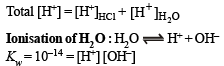Let x be the conc. of [H+] from H2O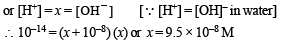[For quadratic equation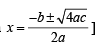∴  Total [H+] = 10–8 + 9.5 × 10–8
= 10.5 × 10–8 or  pH
= – log (10.5 × 10–8) = 6.98
It is between 6 and 7.

QUESTION: 4

The oxidation of SO2 by O2 to SO3 is an exothermic reaction. The yield of SO3 will be maximum if

Solution:

TIPS/Formulae :
(i) According is Le-Chateliers principle, exothermic, reactions are favoured at low temperature.
(ii) According to Le-Chateliers principle, the reaction in which n < 0, are favoured at high pressure.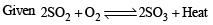∵ It is exothermic reaction
∴Yield of SO3 is maximum at low temperature
n = 2 – 3= – 1 or n < 0
∴ Yield of SO3 is maximum at high pressure.

QUESTION: 5

For the reaction :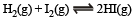the equilibrium constant Kp changes with

Solution:

Only temperature affects the equilibrium constant. Since here ΔH = 2 – 2 = 0 , so there is no change in Kp when total pressure changes.

QUESTION: 6

Of the given anions, the strongest Bronsted base is

Solution:

TIPS/Formulae :

(i) Lower the oxidation state of central atom, weaker will be oxy acid.
(ii) Weaker the acid, stronger will be its conjugate base.
Oxidation state of Cl in HClO is + 1, in HClO2 is + 3, in HClO3 is + 5, and in HClO4 is + 7
∴ HClO is the weakest acid and so its conjugate base ClO is the strongest Bronsted base.

QUESTION: 7

At 90ºC, pure water has [H3O+] 10–6 mole litre–1. What is the value of Kw at 90ºC?

Solution:

For pure water,  [H3O+] = [OH]
⇒ Kw = 10–6 × 10–6 = 10–12

QUESTION: 8

The precipitate of

CaF2(Ksp = 1.7 × 10–10)

is obtained when equal volumes of the following are mixed

Solution:

TIPS/Formulae :
For precipitation to occur ionic product > solubility products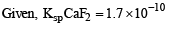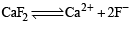Ionic product of C aF2 = [Ca2+] [F-]2 Calculate I.P. in each case

(a) I.P. of CaF2 = (10–4) × (10–4)2  = 10–12
(b) I.P. of CaF2 = (10–2) × (10–3)2  = 10–8
(c) I.P. of CaF2  =  (10–5) × (10–3)2  = 10–11
(d) I.P. of CaF2 =  (10–3) × (10–5)2  = 10–13

∵ I.P > solubility in choice (b) only..
∴ ppt of CaF2 is obtained in case of choice (b) only..

QUESTION: 9

A liquid is in equilibrium with its vapour at its boiling point. On the average, the molecules in the two phases have equal :

Solution:

Vapours and liquid are at the same temperature.

QUESTION: 10

Pure ammonia is placed in a vessel at a temperature where its dissociation constant (a) is appreciable. At equilibrium :

Solution:

Statement (a) is correct and the rest statements are wrong. Kp depends only on temperature hence at constant temp. Kp will not change.

QUESTION: 11

A certain buffer solution contains equal concentration of X and HX. The Kb for X is 10–10. The pH of the buffer is :

Solution:

For a basic buffer,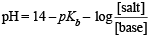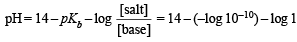⇒ pH = 4

QUESTION: 12

A certain weak acid has a dissociation constant of 1.0 × 10–4. The equilibrium constant for its reaction with a strong base is :

Solution:

TIPS/Formulae :

The equilibrium constant for the nuetralization of a weak acid with a strong base is given by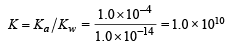QUESTION: 13

An example of a reversible reaction is :

Solution:

As all the reactants and products are present in aqueous form in (d) so  it is a reversible reaction. In others either solid or gas is generated which is insoluble or volatile and hence makes the reaction unidirectional.

QUESTION: 14

The best indicator for detection of end point in titration of a weak acid and a strong base is :

Solution:

TIPS/Formulae :

The pH of the solution at the equivalence point will be greater than 7 due to salt hydrolysis. So an indicator giving colour in basic medium will be suitable.
Phenolphthalein is a good indicator if the base is strong because strong base immediately changes the pH at end point.

QUESTION: 15

The conjugate acid of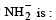Solution:

Base + H+ → (conjugate acid) -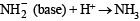(conjugate acid)

QUESTION: 16

The compound that is not a Lewis acid is :

Solution:

NOTE : Electron acceptors or elements having incomplete octet are Lewis acids.
(i) BF3 (B has 6 e in valance shell), AlCl3 (Al has 6 electrons in valance shell), BeCl2 (Be has 4 e in valance shell) are electron defecient compounds and hence Lewis acids.
(ii) SnCl4 has complete octet so it is Lewis base.

QUESTION: 17

The compound insoluble in acetic acid is :

Solution:

CaO, CaCO3 and Ca(OH)2 dissolve in CH3COOH due to formation of  (CH3COO)2Ca. But CaC2O4 does not dissolve as CH3COO is a stronger conjugate base than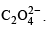QUESTION: 18

The compound whose 0.1 M solution is basic is :

Solution:

(a) is a neutral solution due to both cationic and anionic hydrolysis (Ka = Kb = 1.8×10–5); (b) is acidic solution due to cationic hydrolysis; (c) is acidic solution due to cationic hydrolysis; (d) is basic solution due to anionic hydrolysis.
Alternate solution.

QUESTION: 19

When equal volumes of the following solutions are mixed, precipitation of AgCl (Ksp = 1.8×10–10) will occur only with

Solution:

For a precipitation to occur Solubility product < Ionic product
Given  Ksp = 1.8 × 10–10
Calculating ionic products in each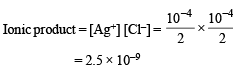which is greater than Ksp (1.8 × 10–10).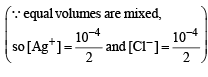QUESTION: 20

The pKa of acetylsalicyclic and (aspirin) is 3.5. The pH of gastric juice in human stomach is about 2-3 and the pH in the small intestine is about 8. Aspirin will be

Solution:

TIPS/Formulae :
In acidic medium weak acids are unionized due to common ion effect and they are completely ionised in alkaline medium.
Aspirin (or acetyl salicylic acid) is unionised in stomach (where pH is 2-3 ) and is completely ionised in small intestine (when pH is 8)

QUESTION: 21

Which one of the following is the strongest acid?

Solution:

TIPS/Formulae :
(i) Higher the electronegatively of central atom higher will be the acidic strength.
(ii) In case of same atom higher the value of oxidation state of the metal, higher will be its acidic strength.
The  electronegativity of Cl > S.
Oxidation no. of Cl in ClO3(OH) = + 7 Oxidation no. of  Cl in ClO2(OH) = + 5 Oxidation no. of S in SO(OH)2 = + 4 Oxidation no. of  S in SO2(OH)2 = + 6

∴ ClO3(OH) is the strongest acid.

QUESTION: 22

Amongst the following hydroxides, the one which has the lowest value of Ksp at ordinary temperature (about 25ºC) is

Solution:

NOTE : In case of alkaline earth hydroxides solubility increases on moving down the group.
Be(OH)2 has lowest solubility and hence lowest solubility product. [Be at tip of the group]

QUESTION: 23

The reaction which proceeds in the forward direction is

Solution:

Due to absence of hydrolysation of FeCl3 backward reaction will not take place.

QUESTION: 24

The following equilibrium in established when hydrogen chloride is dissolved in acetic acid.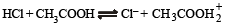The set that characterises the conjugate acid-base pairs is

Solution:

Since HCl is stronger than CH3COOH hence acts as acid. On the other hand Cl is a stronger base than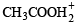and is the conjugate base of HCl.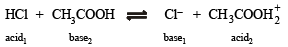QUESTION: 25

Which of the following solutions will have pH close to 1.0?

Solution:

(a) It is not correct answer because 100 ml M/10 HCl will completely neutralise 100 ml M/10 NaOH and the solution will be neutral.
(b) After neutralisation resultant solution will be acidic due to presence of excess of HCl.
(c) After netralisation resultant solution will be basic due to presence of excess of NaOH.
(d) M. eq. of HCl = 75 N/5 = 15 Meq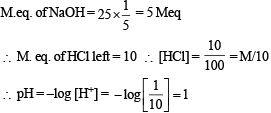QUESTION: 26

The degree of dissociation of water at 25ºC is 1.9 × 10–7% and density is 1.0 g cm–3. The ionic constant for water is :

Solution:

TIPS/Formulae :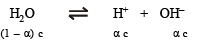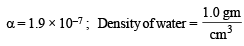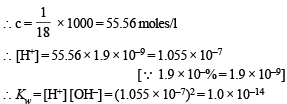QUESTION: 27

Which one is more acidic in aqueous solution

Solution:

Salts of weak base and strong acid get hydrolysed in aqueous solution forming an acidic solution.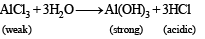QUESTION: 28

The following acids have been arranged in the order of decreasing acid strength. Identify the correct order.

ClOH (I),   BrOH(II),  IOH(III)

Solution:

Among  oxyacids of the same type formed by different elements, acidic nature increases with increasing electronegativity. In general, the strength of oxyacids decreases as we go down the family in the periodic table.

HOCl  (I)     >    HOBr  (II)    >    HOI (III)
[In halogen groups Cl is above Br and I]

QUESTION: 29

The pH of 0.1 M solution of the following salts increases in the order.

Solution:

The characteristics of the given solutions are:
NaCl – neutral solution
NH4Cl – slightly acidic due to the following reaction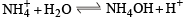NaCN – slightly alkaline due to the following reaction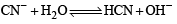HCl – highly acidic
The pH of the solution will follow the order highly acidic < slightly acidic < neutral < slightly alkaline i.e.
HCl < NH4Cl < NaCl < NaCN

QUESTION: 30

For the chemical reaction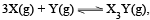the amount of X3Y at equilibrium is affected by

Solution:

The given reaction will be exothermic in nature due to the formation of three  X - Y bonds from the gaseous atoms. The reaction is also accompanied with the decrease in the gaseous species (i.e. Dn is negative).
Hence, the reaction will be affected by both temperature and pressure. The use of catalyst does not affect the equilibrium concentrations of the species in the chemical reaction.

QUESTION: 31

For the reversible reaction,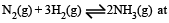500°C, the value of Kp is 1.44 x10-5 when partial pressure is measured in atmospheres. The corresponding value of Kc, with concentration in mole litre–1, is

Solution:

Kb = Kc .( RT)Δn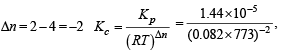(R in L.atm.K–1 mole–1).

QUESTION: 32

When two reactants, A & B are mixed to give products C & D, the reaction quotient Q, at the initial stages of the reaction

Solution:

At initial stage of reaction, concentration of each product will increase and hence Q will increase.

QUESTION: 33

For a sparingly soluble salt ApBq, the relationship of its solubility product (LS) with its solubility (S) is

Solution: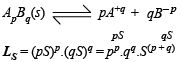QUESTION: 34

The set with correct order of acidity is

Solution:

TIPS/Formulae : For oxyacids containing similar central atom, the acid strength increases with the increase in the number of oxygen atom attached to the central atom and not attached to any other atom.
TIPS/Formulae : Higher the oxidation number of the central atom, higher is the acidity of the species. Thus acidity  follows the order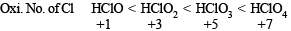QUESTION: 35

At constant temperature, the equilibrium constant (Kp) for the decomposition reaction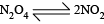is expressed by Kp = (4x2P)/(1-x2), where P = pressure, x = extent of decomposition. Which one of the following statements is true?

Solution:

At constant temperature Kp pressure constant.
With change of pressure, x will change in such a way that Kp remains a constant.

QUESTION: 36

Consider the following equilibrium in a closed container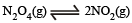At a fixed temperature, the volume of the reaction container is halved. For this change, which of the following statements holds true regarding the equilibrium constant (Kp) and degree of dissociation (α)?

Solution:

TIPS/Formulae :

At constant temperature Kp or Kc remains constant.
For the equilibria :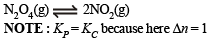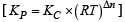Since temperature is constant so Kc or Kp will remain constant. Further since volume is halved, the pressure will be doubled so a will decrease so as to maintain the constancy of Kc or Kp.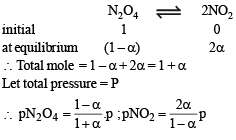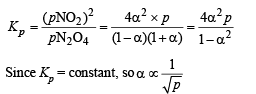So when volume is halved, pressure gets doubled and thus α will decrease.

QUESTION: 37

A weak acid HX has the dissociation constant 1 × 10–5 M. It forms a salt NaX on reaction with alkali. The percentage hydrolysis of 0.1 M solution of NaX is

Solution: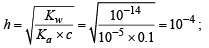Hence, % hydrolysis = 10–4 × 100 = 0.01

QUESTION: 38

A 0.004 M solution of Na2SO4 is isotonic with 0.010 M solution of glucose at same temperature. The  percentage dissociation of Na2SO4 is

Solution:

NOTE : For isotonic solutions, osmotic pressure is same.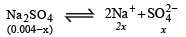Since both solutions are isotonic, therefore, 0.004 + 2x = 0.01 ; x = 3 × 10–3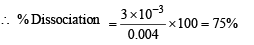Alternate eqn. Total no. of moles = 0.004 × x + 2x + x

QUESTION: 39

0.1 mole of CH3NH2 (Kb = 5 x10–4)is mixed with 0.08 mole of HCl and diluted to one litre. What will be the H+ concentration in the solution?

Solution:

NOTE : For basic buffer pH is more than 7.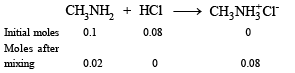As it is a basic buffer solution.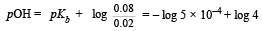=  3.30  +  0.602  = 3.902
pH  = 14 – 3.902 = 10.09; [H+]  = 7.99 × 10–11 ≈ 8 ×10–11 M

QUESTION: 40

The Haber ’s process for the formation of NH3 at 298K is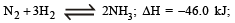Wh ich of the following is the correct statement

Solution:

In a reversible reaction, catalyst speeds up both the forward and backward reactions to the same extent, so (c) is wrong. At equilibrium,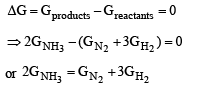QUESTION: 41

2.5 ml of (2/5) M weak monoacidic base (Kb = 1 × 10–12 at 25°) is titrated (2/15) M HCl in water at 25°C. The concentration  of H+ at equivalence point is (Kw = 1 × 10–14 at 25°C)

Solution:

Let the weak manoacidic base be BOH, then the reaction that occurs during titration is

BOH + HCl → BCl + H2O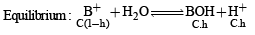Using the normality equation,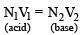Substituting various given values, we get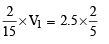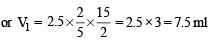Then the concentration of BCl in resulting solution is given by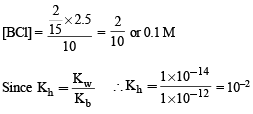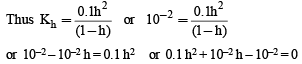(Solving this quadratic equation for h, we get)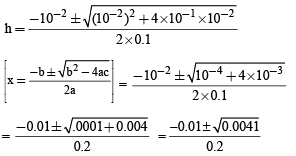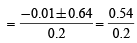[Neglecting the negative term]

= 0.27
∴ [H+] = C. h  = 0.1 × 0.27  = 2.7 × 10–2 M

Thus the correct answer is [d].

QUESTION: 42

Solubility product constant (Ksp) of salts of types MX, MX2 and M3X at temperature T are 4.0 × 10–8, 3.2 × 10–14 & 2.7 × 10–15, respectively. Solubilities (mol dm–3) of the salts at temperature 'T' are in the order –

Solution: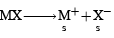(Where s is the solubility)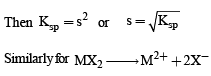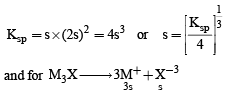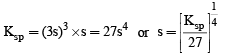From the given values of Ksp for MX, MX2 and M3X, we can find the solubilities of those salts at temperature, T.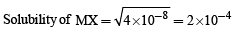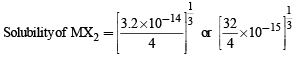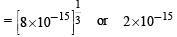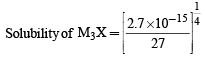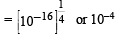Thus the solubilities are in the order MX > M3 X> MX2 i.e the correct anser is (d).